Скачать презентацию Chapter 11 Monopoly We are now back

65104b3fedd45ec7b4ddf97a6e5c34ed.ppt

• Количество слайдов: 24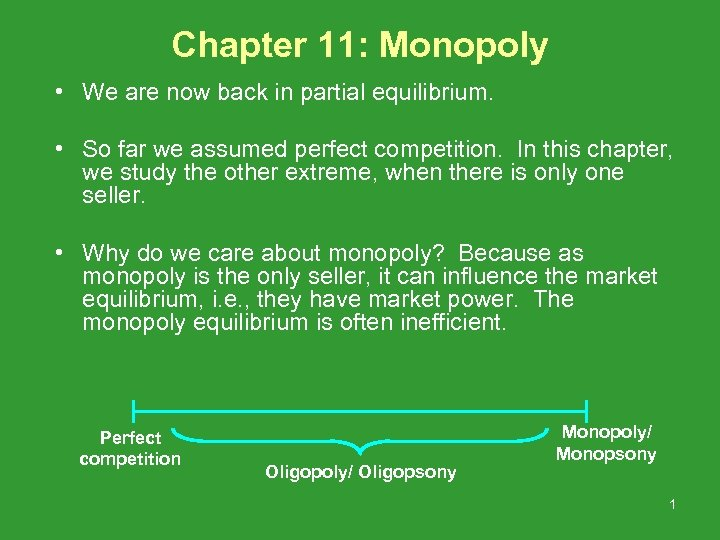Chapter 11: Monopoly • We are now back in partial equilibrium. • So far we assumed perfect competition. In this chapter, we study the other extreme, when there is only one seller. • Why do we care about monopoly? Because as monopoly is the only seller, it can influence the market equilibrium, i. e. , they have market power. The monopoly equilibrium is often inefficient. Perfect competition Oligopoly/ Oligopsony Monopoly/ Monopsony 1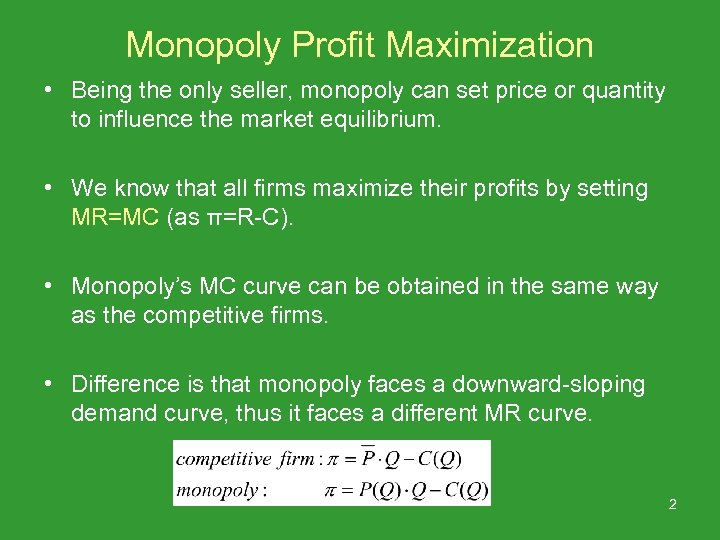Monopoly Profit Maximization • Being the only seller, monopoly can set price or quantity to influence the market equilibrium. • We know that all firms maximize their profits by setting MR=MC (as π=R-C). • Monopoly’s MC curve can be obtained in the same way as the competitive firms. • Difference is that monopoly faces a downward-sloping demand curve, thus it faces a different MR curve. 2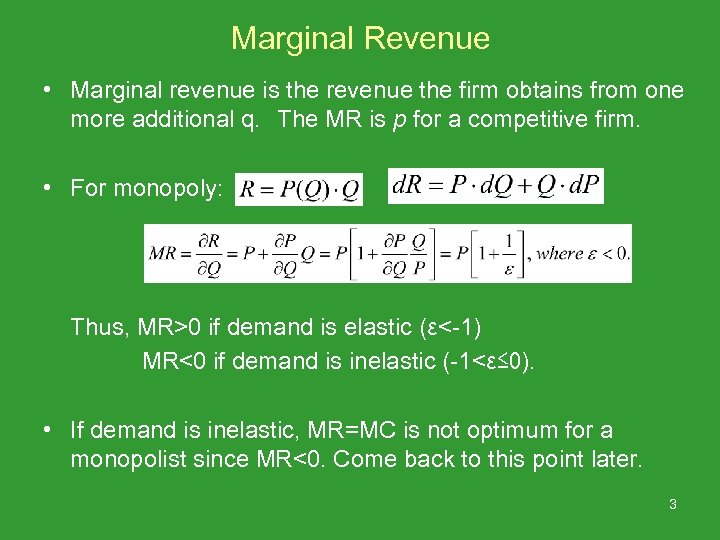Marginal Revenue • Marginal revenue is the revenue the firm obtains from one more additional q. 　The MR is p for a competitive firm. • For monopoly: Thus, MR>0 if demand is elastic (ε<-1) MR<0 if demand is inelastic (-1<ε≤ 0). • If demand is inelastic, MR=MC is not optimum for a monopolist since MR<0. Come back to this point later. 3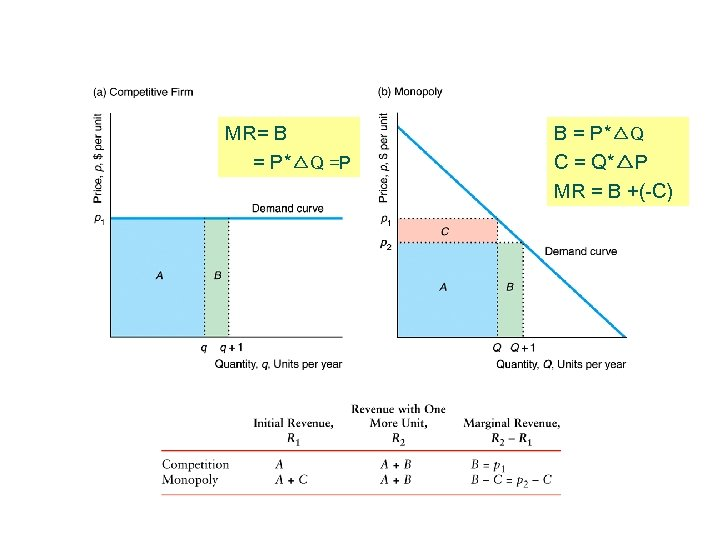MR= B = P*△Q =P B = P*△Q C = Q*△P MR = B +(-C) 4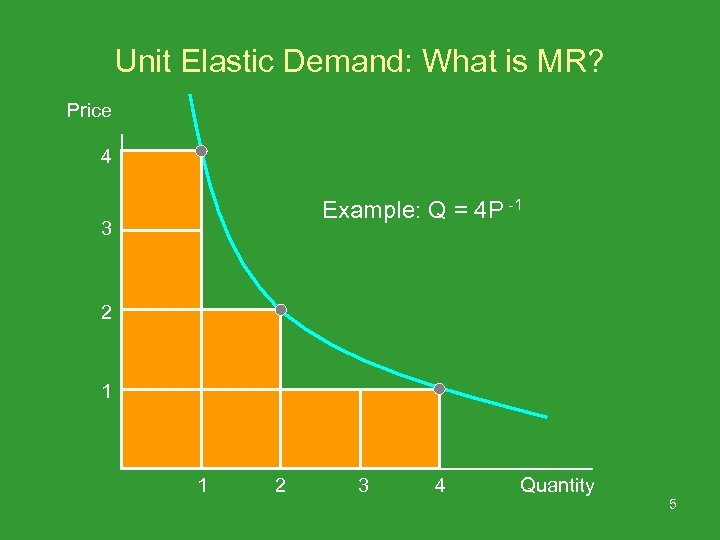Unit Elastic Demand: What is MR? Price 4 Example: Q = 4 P -1 3 2 1 1 2 3 4 Quantity 5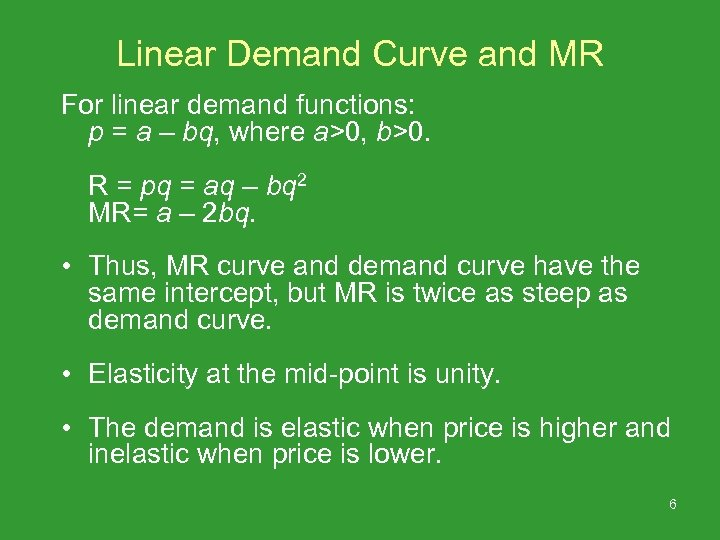Linear Demand Curve and MR For linear demand functions: p = a – bq, where a>0, b>0. R = pq = aq – bq 2 MR= a – 2 bq. • Thus, MR curve and demand curve have the same intercept, but MR is twice as steep as demand curve. • Elasticity at the mid-point is unity. • The demand is elastic when price is higher and inelastic when price is lower. 6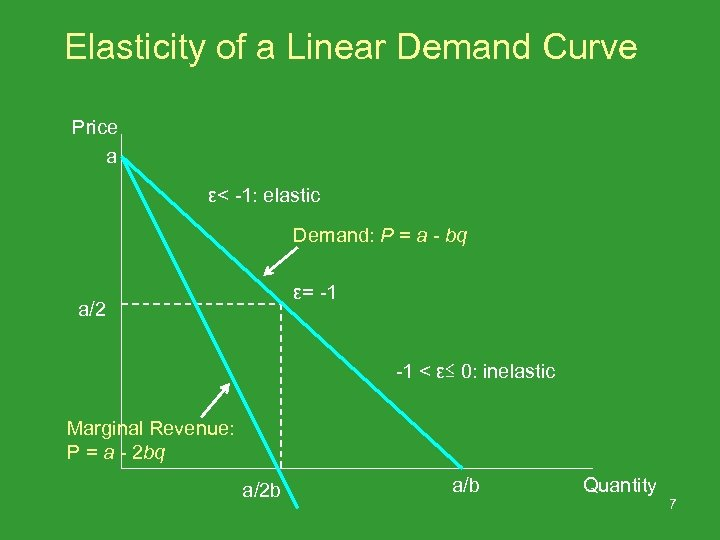Elasticity of a Linear Demand Curve Price a ε< -1: elastic Demand: P = a - bq ε= -1 a/2 -1 < ε≤ 0: inelastic Marginal Revenue: P = a - 2 bq a/2 b a/b Quantity 7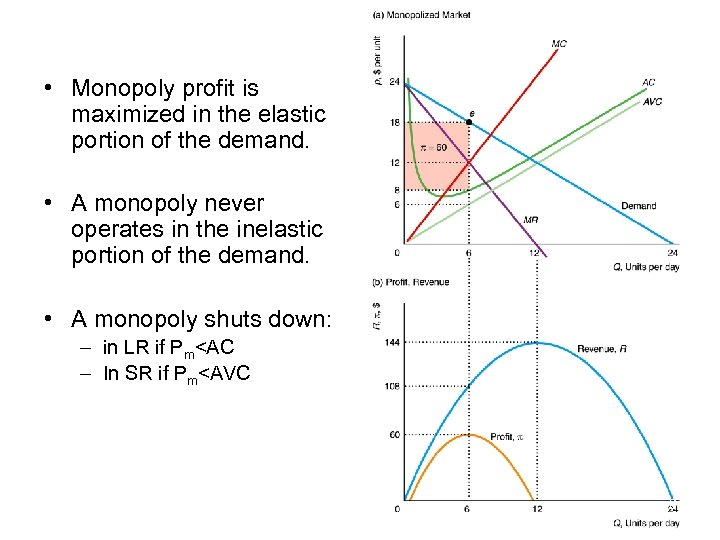• Monopoly profit is maximized in the elastic portion of the demand. • A monopoly never operates in the inelastic portion of the demand. • A monopoly shuts down: – in LR if Pm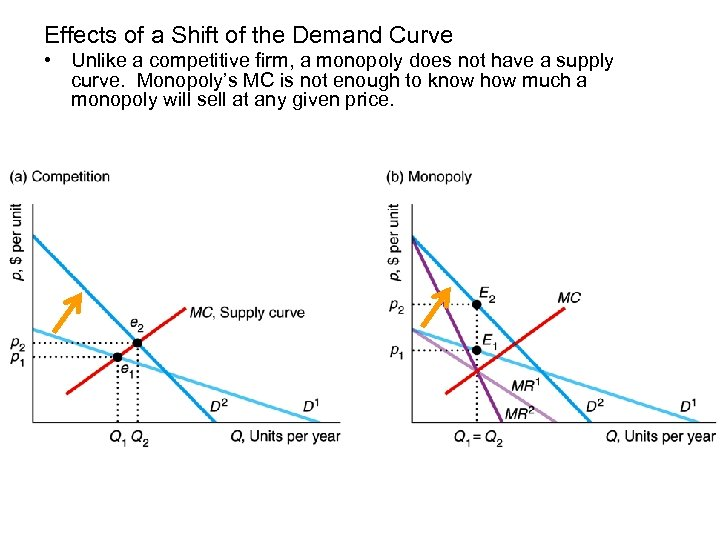Effects of a Shift of the Demand Curve • Unlike a competitive firm, a monopoly does not have a supply curve. Monopoly’s MC is not enough to know how much a monopoly will sell at any given price. 9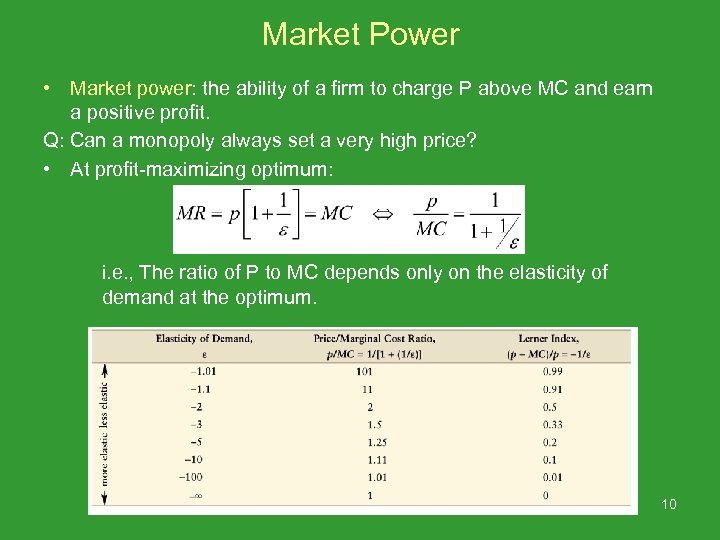Market Power • Market power: the ability of a firm to charge P above MC and earn a positive profit. Q: Can a monopoly always set a very high price? • At profit-maximizing optimum: i. e. , The ratio of P to MC depends only on the elasticity of demand at the optimum. 10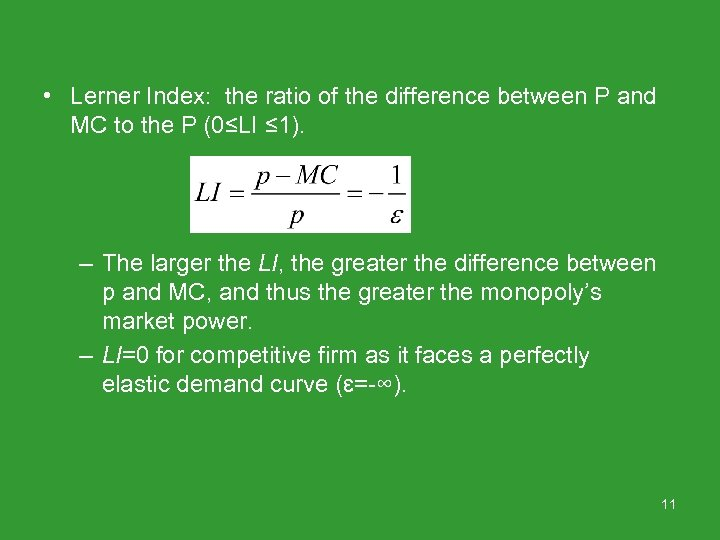• Lerner Index: the ratio of the difference between P and MC to the P (0≤LI ≤ 1). – The larger the LI, the greater the difference between p and MC, and thus the greater the monopoly’s market power. – LI=0 for competitive firm as it faces a perfectly elastic demand curve (ɛ=-∞). 11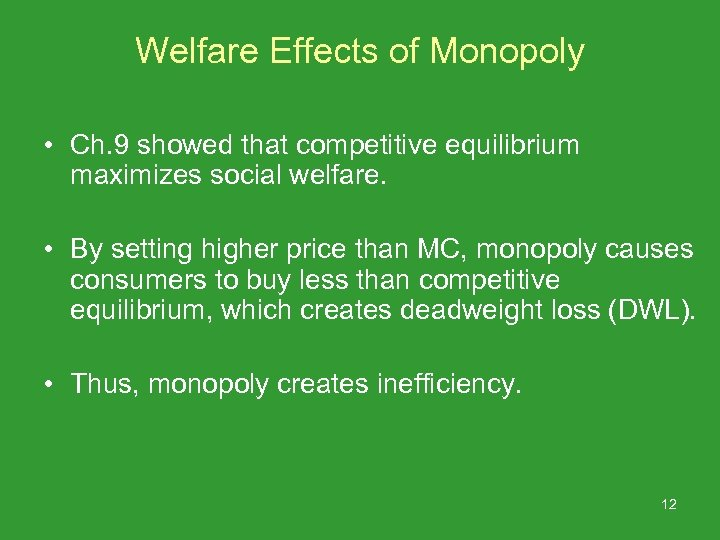Welfare Effects of Monopoly • Ch. 9 showed that competitive equilibrium maximizes social welfare. • By setting higher price than MC, monopoly causes consumers to buy less than competitive equilibrium, which creates deadweight loss (DWL). • Thus, monopoly creates inefficiency. 12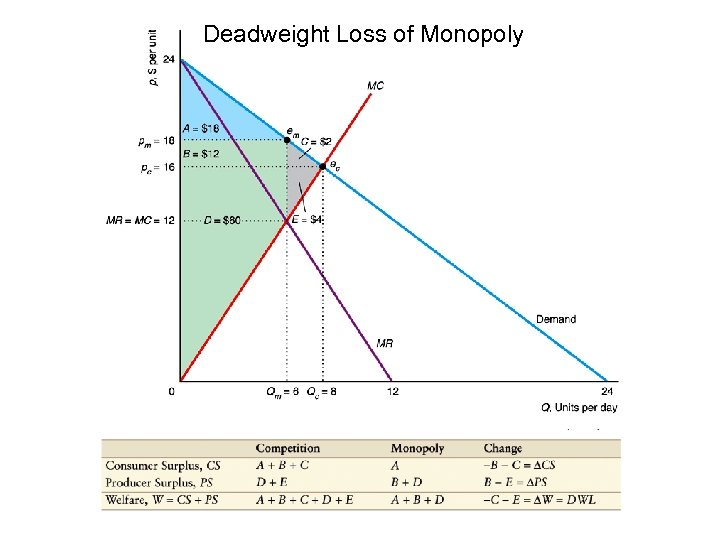Deadweight Loss of Monopoly 13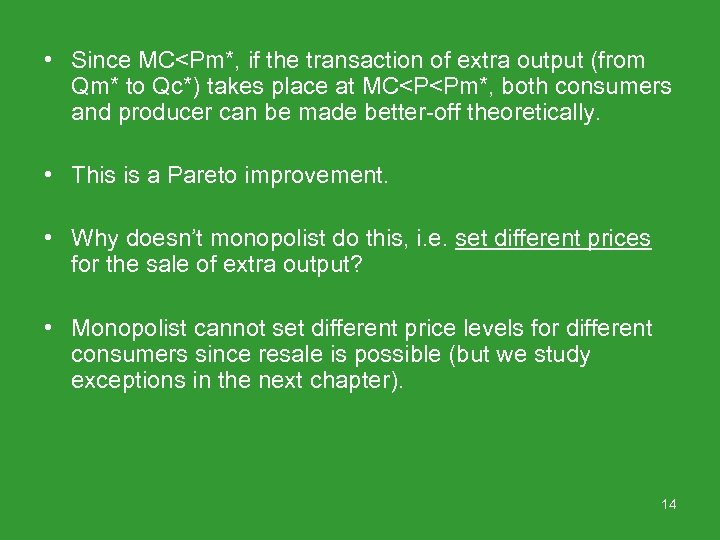• Since MC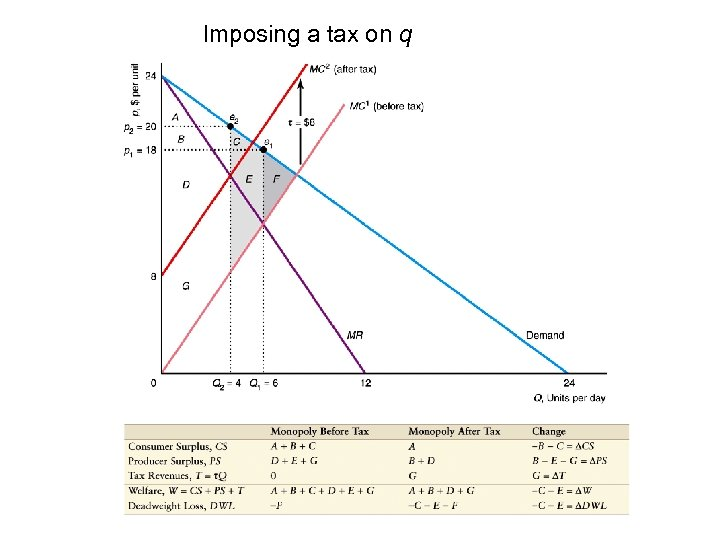Imposing a tax on q 15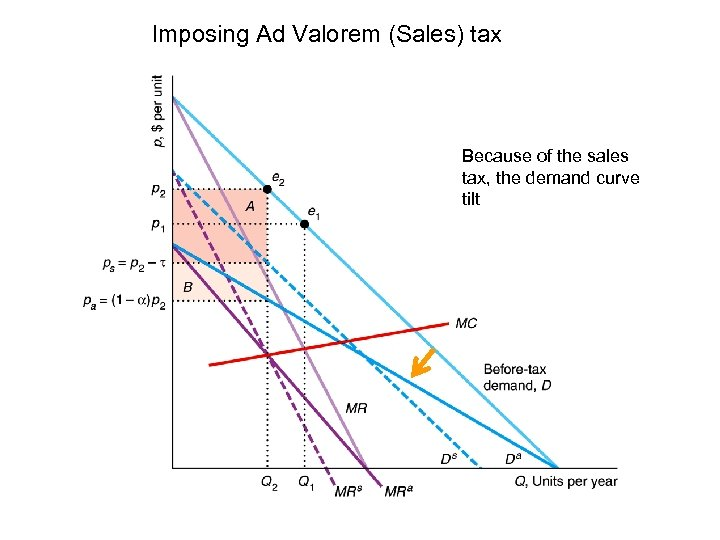Imposing Ad Valorem (Sales) tax Because of the sales tax, the demand curve tilt 16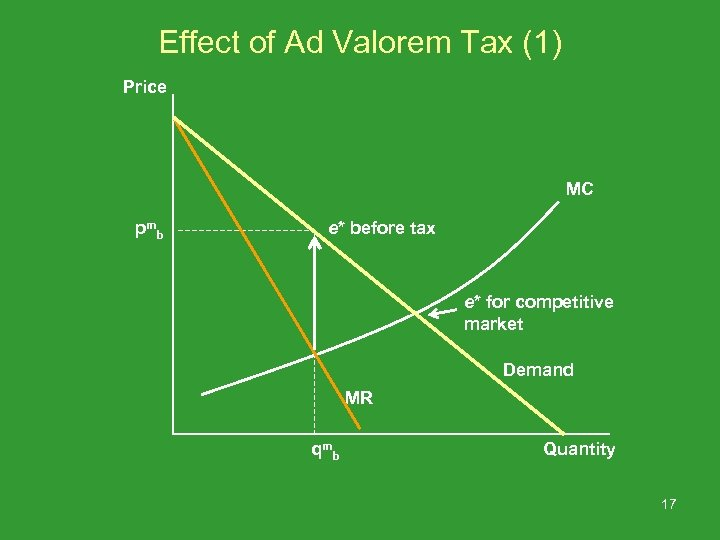Effect of Ad Valorem Tax (1) Price MC pm b e* before tax e* for competitive market Demand MR qm b Quantity 17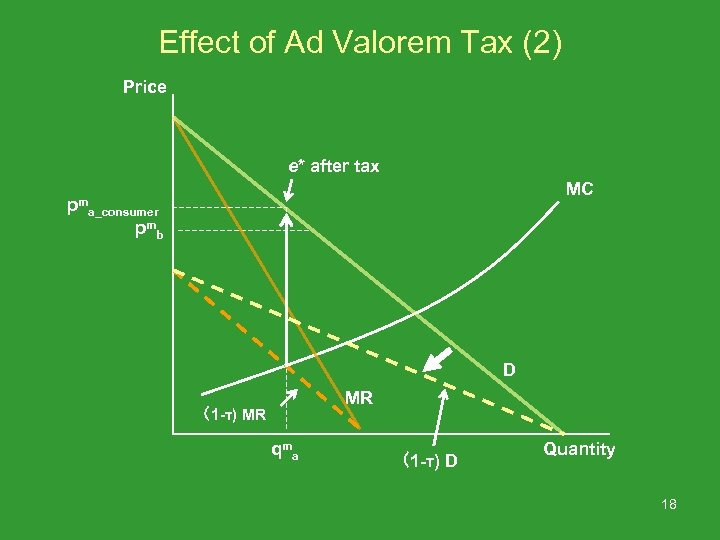Effect of Ad Valorem Tax (2) Price e* after tax pm MC a_consumer pm b D MR （1 -τ) MR qm a （1 -τ) D Quantity 18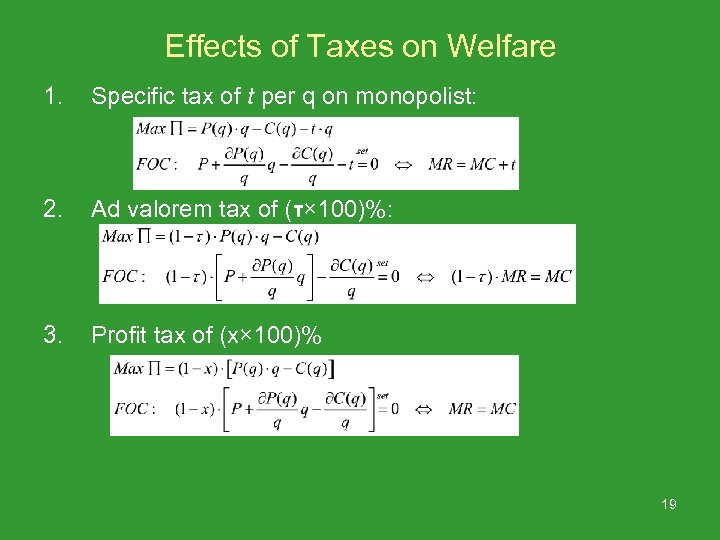Effects of Taxes on Welfare 1. Specific tax of t per q on monopolist: 2. Ad valorem tax of (τ× 100)%: 3. Profit tax of (x× 100)% 19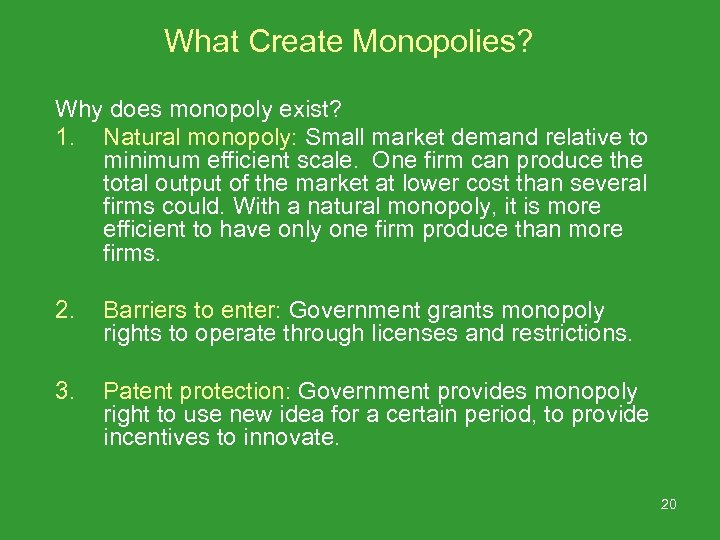What Create Monopolies? Why does monopoly exist? 1. Natural monopoly: Small market demand relative to minimum efficient scale. One firm can produce the total output of the market at lower cost than several firms could. With a natural monopoly, it is more efficient to have only one firm produce than more firms. 2. Barriers to enter: Government grants monopoly rights to operate through licenses and restrictions. 3. Patent protection: Government provides monopoly right to use new idea for a certain period, to provide incentives to innovate. 20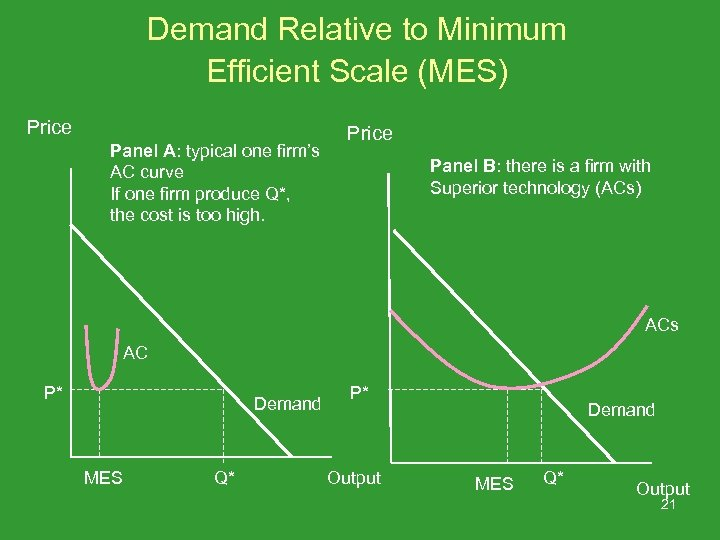Demand Relative to Minimum Efficient Scale (MES) Price Panel A: typical one firm’s AC curve If one firm produce Q*, the cost is too high. Price Panel B: there is a firm with Superior technology (ACs) ACs AC P* Demand MES Q* P* Output Demand MES Q* Output 21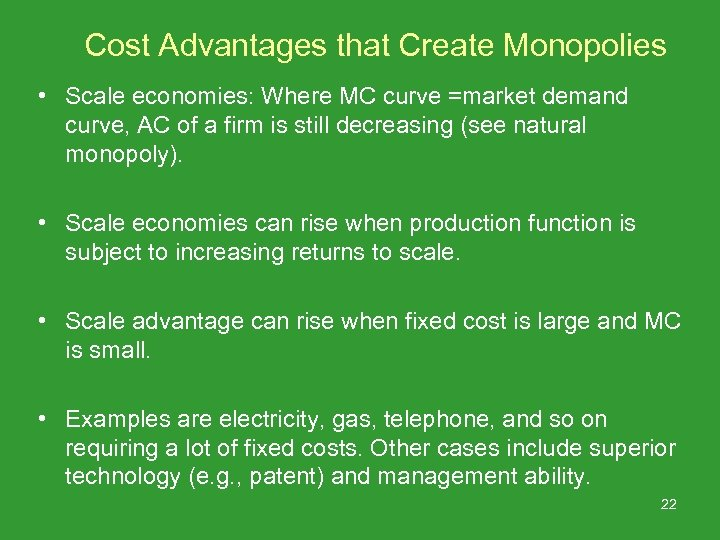Cost Advantages that Create Monopolies • Scale economies: Where MC curve =market demand curve, AC of a firm is still decreasing (see natural monopoly). • Scale economies can rise when production function is subject to increasing returns to scale. • Scale advantage can rise when fixed cost is large and MC is small. • Examples are electricity, gas, telephone, and so on requiring a lot of fixed costs. Other cases include superior technology (e. g. , patent) and management ability. 22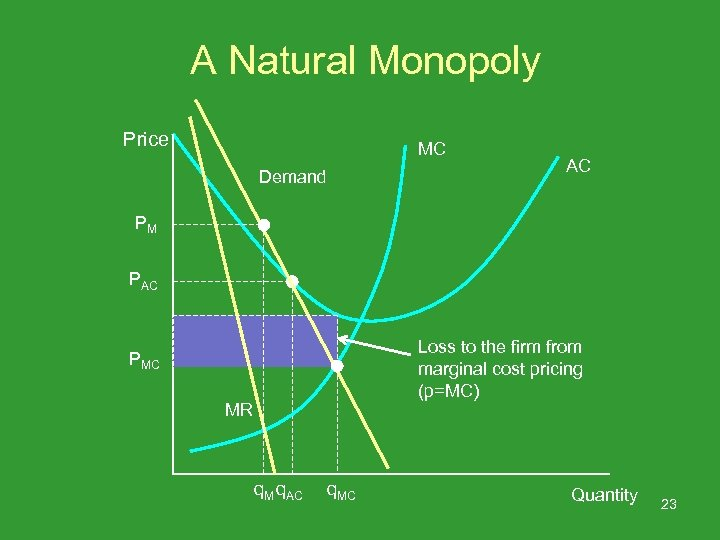A Natural Monopoly Price MC Demand AC PM PAC Loss to the firm from marginal cost pricing (p=MC) PMC MR q. M q. AC q. MC Quantity 23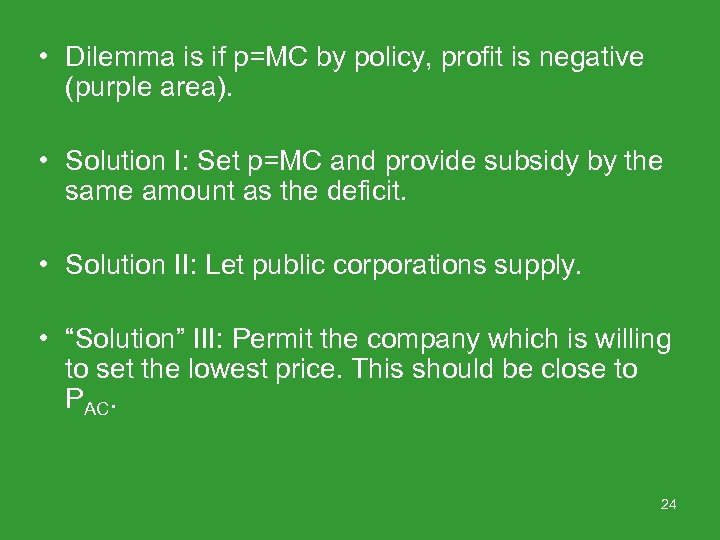• Dilemma is if p=MC by policy, profit is negative (purple area). • Solution I: Set p=MC and provide subsidy by the same amount as the deficit. • Solution II: Let public corporations supply. • “Solution” III: Permit the company which is willing to set the lowest price. This should be close to PAC. 24